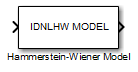# Hammerstein-Wiener Model

Simulate Hammerstein-Wiener model in Simulink software

• Library:
• System Identification Toolbox / Models## Description

The Hammerstein-Wiener Model block simulates the output of a Hammerstein-Wiener model using time-domain input data. The model is an `idnlhw` model that you previously estimated or constructed in the MATLAB® workspace. You specify initial conditions for the simulation as one of the following:

• Zero for all states

• Initial state vector representing the initial states of the linear block

For information about the structure of a Hammerstein-Wiener model, see What are Hammerstein-Wiener Models?.

## Ports

### Input

expand all

Simulation input data, specified as a scalar for a single-input model. The data must be time-domain data. For multi-input models, specify the input as an Nu-element vector, where Nu is the number of inputs. For example, you can use a Vector Concatenate block to concatenate scalar signals into a vector signal.

### Note

Do not use a Bus Creator or Mux block to produce the vector signal.

Data Types: `double`

### Output

expand all

Simulated output from Hammerstein-Wiener model, returned as a scalar for a single-output model and as an Ny-element vector for a model with Ny outputs.

Data Types: `double`

## Parameters

expand all

Hammerstein-Wiener model to be simulated, specified as an `idnlhw` object. You previously estimate or construct the `idnlhw` model in the MATLAB workspace.

The states of a Hammerstein-Wiener model correspond to the states of the embedded linear `idpoly` or `idss` model. For more information about the states, see the `idnlhw` reference page. You specify `Initial conditions` as one of the following:

• `Zero` — Specifies zero initial state values, which correspond to a simulation starting from a state of rest.

• `State values` — You specify the state values in Specify a vector of state values. Specify the states as a vector of length equal to the number of states in the model.

If you do not know the initial states, you can estimate these states as follows:

• To simulate the model around a given input level when you do not know the corresponding output level, you can estimate the equilibrium state values using the `findop` command.

For example, to simulate a model `M` about a steady-state point where the input is `1` and the output is unknown, you can specify the initial state values as `X0`, where:

`X0 = findop(M,'steady',1,NaN)`
• To estimate the initial states that provide a best fit between measured data and the simulated response of the model for the same input, use the `findstates` command.

For example, to compute initial states such that the response of the model `M` matches the simulated output data in the data set `z`, specify `X0`, such that:

`X0 = findstates(M,z)`

## Extended Capabilities

### Topics

#### Introduced in R2008a

##### SupportGet trial now